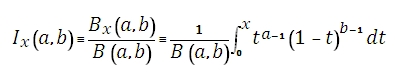# SQL Server incomplete beta function

Updated: 9 August 2010

Use BETAI to calculate the incomplete beta function. The incomplete beta function is defined by:Syntax
SELECT [wctStatistics].[wct].[BETAI] (
<@A, float,>
,<@B, float,>
,<@X, float,>)
Arguments
@A
is any real number greater than or equal to zero. @A is an expression of type float or of a type that can be implicitly converted to float@A is the lower bound to the distribution. If you enter NULL, @A defaults to zero
@B
is any real number greater than or equal to zero. @B is an expression of type float or of a type that can be implicitly converted to float.   @B is the upper bound to the distribution. If you enter NULL @B defaults to to zero.
@X
is any real number greater than or equal to zero and less than or equal to one. @X is an expression of type float or of a type that can be implicitly converted to float
Return Types
float
Remarks
·         If @A ≤ 0 or @B ≤ 0, BETAI returns an error.
·         If @X < 0 or @X > 1, BETAI returns an error.
·         @X = 0 or @X = 1, BETAI = @X
·         BETAI(@A, @B, @X) = BETADIST(@X, @A, @B, 0, 1)
Examples

select
wct.BETAI(1, 5, .45)

This produces the following result

----------------------
0.9496715625

(1 row(s) affected)

This produces the following result

----------------------
0.9496715625

(1 row(s) affected)

### SupportCopyright 2008-2022 Westclintech LLC         Privacy Policy        Terms of Service